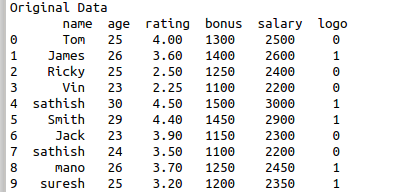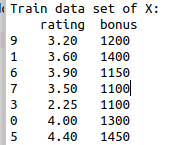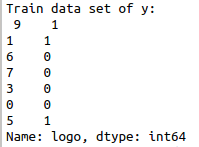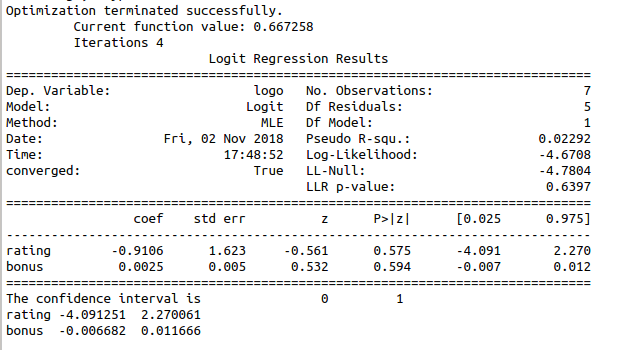• #5, First Floor, 4th Street Dr. Subbarayan Nagar Kodambakkam, Chennai-600 024 Landmark : Samiyar Madam
• pro@slogix.in
• +91- 81240 01111

#### Social List

##### How to build logistic regression model for train data set using python?
###### Description

To build a logistic regression model for the given data set in python.

###### Process

Import libraries.

Define X and y variables.

Build the logistic regression model.

Split the sample data into training and test data.

Fit the training data into the regression model.

###### Sapmle Code

#import libraries

import statsmodels.api as sm
import pandas as pd
from sklearn.model_selection import train_test_split

Pythonfiles/Employee.csv’)

#creating data frame
df=pd.DataFrame(data)
print(df)

#assigning the independent variable
X = df[[‘rating’,’bonus’]]

#assigning the dependent variable
y = df[‘logo’]

#split data in training and test data
X_train, X_test, y_train, y_test = train_test_split(X, y, test_size=0.3, random_state=0)
print(“Train data set of X:\n”,X_train)
print(“Train data set of y:\n”,y_train)

#build the model
model = sm.Logit(y_train, X_train)

#fit the model
result = model.fit()

#take summary of model
print(result.summary())

#print the confidence interval
print(“The confidence interval is”,result.conf_int())

###### Screenshots